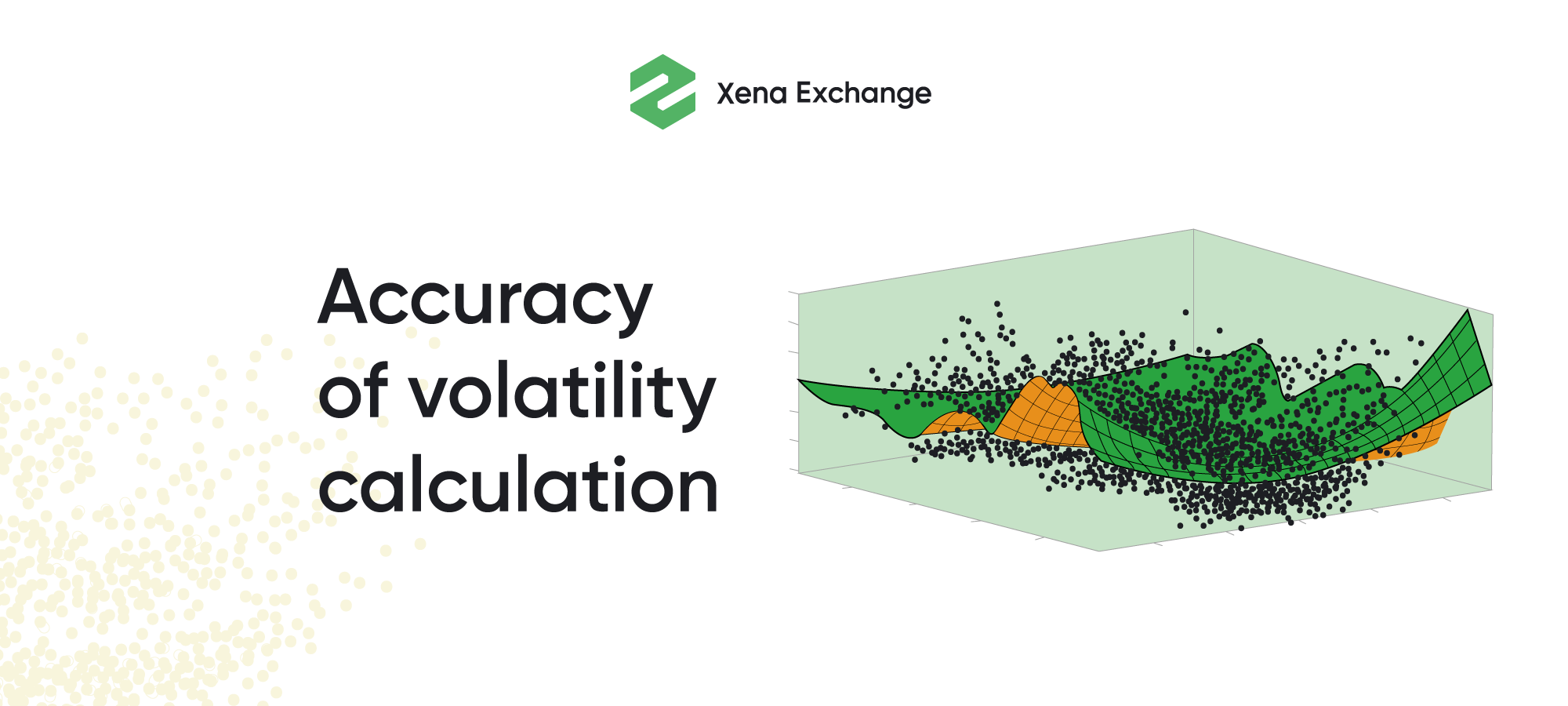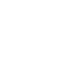# Volatility Index Calculation And Usage In The CryptomarketIn this article, we will talk about the correct and accurate method for calculating volatility in the cryptocurrency market and how this index can help us in an applied sense.
As a rule, all financial market participants have their own view of the market and perceive the market in different paradigms. For example, actors with small capital, in my opinion, tend to perceive the market in terms of profitability. The more trading capital becomes, the less people think about profitability and more about risk. Risk and return are two measures that are directly related. Together, these metrics balance the space of possible actions of each investor, thereby creating a true reality of the financial market. These two metrics, in turn, are very closely related to the concept of volatility, because it is the volatility of the underlying asset that largely determines the action space for each participant in the financial market. In this context, it becomes obvious that volatility is a metric that should always be taken into account. It is especially important to use it for trading algorithms. Let's see how volatility can be measured in the crypto market and how it can be applied.
Accuracy of volatility calculation
Implied volatility is a very fragile concept because its calculations are based on option prices, which in turn are calculated using a formula with its own limitations and assumptions. Therefore, it is important to calculate the volatility as accurately as possible, because every inaccuracy or delay leads to the “butterfly effect”, and the dynamics of the index (especially for a short period of time) becomes chaotic and does not reflect it’s real value.
In an applied sense, an inaccurate volatility is a bad predictor, or, if too many approximations are made, a predictor with a long time-lag. For this reason, within the framework of the calculation methodology, we should take the maximum amount of information about options in order not to miss micro changes. The more options we take into account, the more accurately we describe volatility. The dollar vega of the cumulative portfolio to describe volatility should be equal to 1. This will mean that a 1% change in the portfolio will describe a 1% change in volatility. Let's see how portfolio PnL changes depending on the number of options in the portfolio. The figure below shows that it would be better to take as many options as possible in order to correctly calculate volatility.
Methodology
1. In the first place, we find the target time for which we will calculate the index. The target time is calculated according to the following formula:
• Сurrent time + Number of days to calculate the index [1, 2, 7, 14, 21, 28]
2. We find the option series, the expiration date of which is earlier than our target time. From these series, select the one whose expiration date is closest to the target time.
We repeat the same selection for options that expire after our target time. From them we select options with the nearest expiration date
Two found option series (one with expiration before the target time and one after) will be used to calculate the index.
3. The next step is to find the time difference between the target time and the expiration date in minutes. In this case, expiration occurs at 8:00 UTC
• T = { MCurrent + MSettlement + MOther days } / Minutes in a year
where:
MCurrent - minutes remaining until midnight of the current day
MSettlement - minutes from midnight until 8:00 UTC
MotherDays - total minutes in the days between current day and expiration day
Minutes in a year - is a constant, equal to 525 600
4. In our case, the Risk Free Rate (Rf) is equal to 0. In the traditional calculation of the index we would take the US Treasuries Index as a reference value, but for the cryptocurrency market this value is not entirely correct. In the case of cryptocurrencies, we can extract the Rf from derivatives, but it will be necessary to exclude the risk of the settlement currency. According to the VIX formula, the Rf introduces negligible error into the final result, especially if you take into account the approximate differences of Rf in the traditional market and in the cryptocurrency market. The integration of Rf calculations is very time-consuming work that would be wise to skip.
5. Next, we find the Strike Prices for both series to calculate the forward price. In our case, it will be a strike with the minimum Q-value (bid price + ask price) / 2. For further calculations, we take the Call Price and Put Price on this Strike Price.
* The traditional VIX calculation does not include options that have less than 1 hour left until expiration. In such options liquidity disappears and traders transfer it to the next expiration. In such a situation, when bid and ask are equal to None, we use Market Price (the price of the last filled order) as the value.
6. Next, we calculate the forward index price for each of the two option series:
7. Calculate K0 - the strike price equal to or otherwise immediately below the forward index level, F - for the near- and next term options.
8. Select out-of-the-money put options with Strike Price <= K0. Stop calculation if two None values in a row. Repeat the procedure for call options with Strike Price >= K0.
9. For the selected options calculate ΔKi (half the difference between the strike prices on either side of Ki, where i is the Strike Price). At the upper and lower edges of any given strip of options, ΔKi is simply the difference between Ki and the adjacent strike price.
10. Calculate the value Cumulative Value for both near and long term options:
For one-day near options, the Cumulative Value can be zero at the moments when there is no liquidity in the orderbook. The chart below shows that Cumulative Value decreases linearly towards the expiration date. The nature of the curve allows us to quite easily interpolate missing values. To do this, we take the last 500 values, remove outliers that lie outside the 2-sigma range, and use the obtained poinыts to interpolate values with the least squares method.

11. Calculate squared volatility for both near and next term options:

And values for VIX calculation:
• NTnear = number of minutes to settlement of the near-term options
• NTnext = number of minutes to settlement of the next-term options
• Ni = number of minutes in i [1, 2, 7, 14, 21, 28] days (i x 1,440)
• N365 = number of minutes in a 365-day year (365 x 1,440 = 525,600)
12. Finally, calculate the index:
For a more accurate calculation, you can collect information about options from several major derivatives exchanges and average the results. Technically, it is quite simple to do this, since the APIs of options trading exchanges are quite similar and to collect the necessary data from one exchange, only 2 requests are enough, and somewhere even 1.
As a result, you should get an index similar to the one shown in the picture below. It is a cumulative Bitcoin volatility index compiled from 3 different exchanges. As you can see, values of the chart get more and more variable as the target time decreases.
Usage
First of all, this index is very convenient to trade as a derivative. It helps you skip building complex option strategies, just like variation futures do. Volatility index allows to simply trade volatility as the price of the underlying asset and simplifies the hedging process.
The volatility index can be used for various algorithmic trading tasks, for example, placing a stop loss. In its simplest form, algorithmic traders use the ATR indicator for adaptive placement of a stop loss. ATR is an indicator with a very slow reaction for a price change (especially with a long lookback period). Short-term volatility index should give better results if used for stop-loss placing. You can optionally use the indicator as a predictor, entry signal or a position filter. In any case, strategies with such an indicator can greatly diversify the portfolio.
Also, the volatility index is a great helper to understand the risk associated with crypto investment or algo portfolio. Depending on the parameters of the portfolio, the values of the index can be used to adjust and adaptively change money and risk management.
In this article, we figured out how to accurately calculate the volatility index for crypto and what this index is useful for. If you are interested in this topic and want to discuss your developments, you can write to me directly: https://www.linkedin.com/in/hsergeyf/